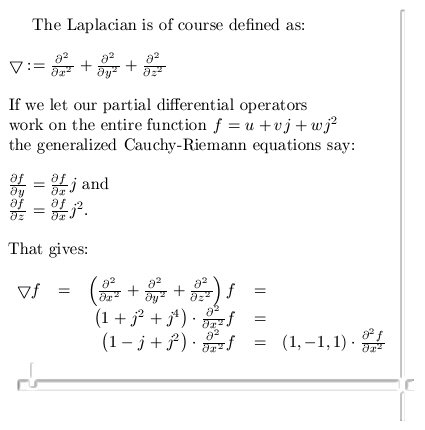# Calculating the Laplacian using the Cauchy-Riemann equations.

Without doubt the Laplacian is a very important differential operator. It plays a major role in for example the classical wave equation and also the Schrödinger wave equation from quantum mechanics.

Now scroll a bit back until you find the post on the Cauchy-Riemann equations, at the end I used the phrase ‘Cauchy-Riemann equations chain rule style’ and this is how we can crack in a very easy way how the Laplacian operates on functions that obey the CR equations on 3D complex numbers.

I have hundreds and hundreds of pages of math stuff on the 3D complex number system and very often I use the number alpha. This number alpha is so important, not only in 3D, that it is worth to post a few posts on them.

For the time being, I just conducted a simple Google search on the phrase ‘3d complex numbers’ in the search detail for pictures. And every time this old teaser picture from the other website pops up:At the end you see that (1, -1, 1), well that is three times alpha.

It is a nice exercise to prove that the square of alpha equals alpha.
So alpha is in the same category as for example numbers like 0 and 1 because if you square those you also get the original number back in return.

After all one squared equals one and zero squared equals zero.

End of this update, till updates.